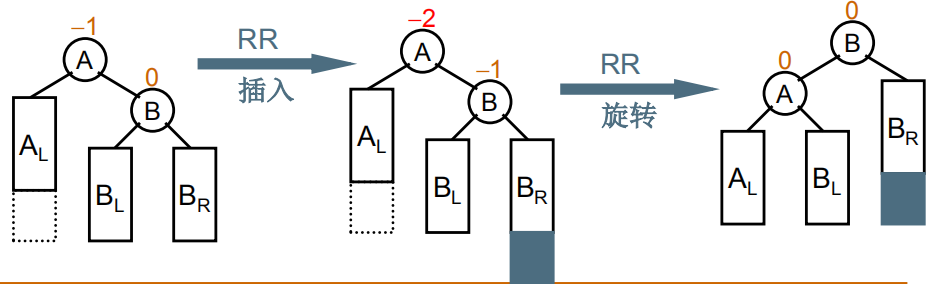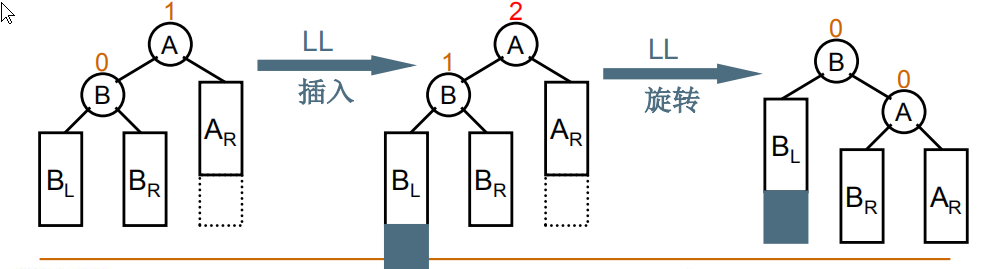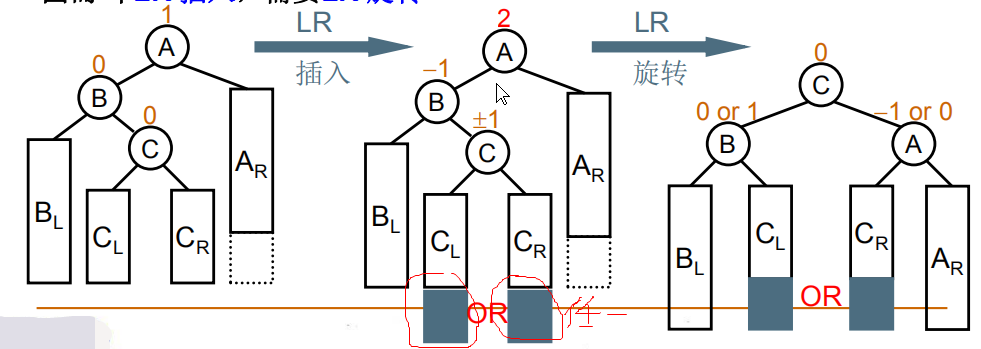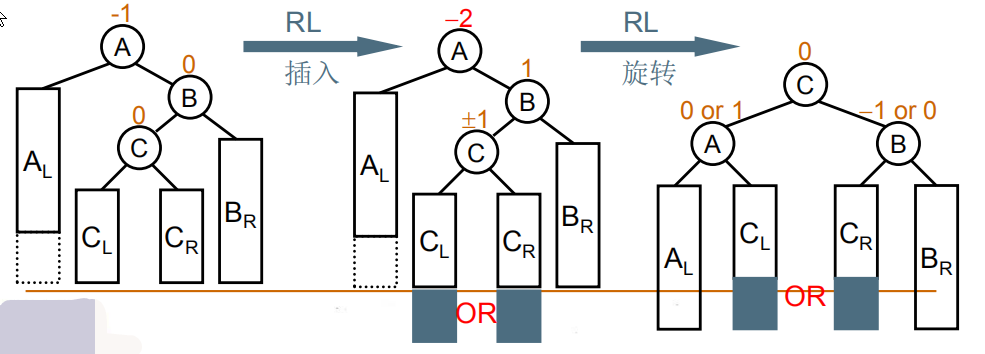# 平衡二叉树插入操作的原理及实现

typedef struct node *AVL;
struct node{
ElementType data;
int height;
AVL left;
AVL right;
}


• 获得节点的高度：
int GetHeight(AVL tree)
{
if(!tree) return 0;
return tree->height;
}

• 更新节点高度：
AVL UpdateHeight(AVL tree)
{
tree->height=max(GetHeight(tree->left),GetHeight(tree->right))+1;
return tree;
}

• 得到节点的平衡因子：
int GetBT(AVL tree)
{
int BT=GetHeight(tree->left)-GetHeight(tree->right);//不用绝对值是因为方便判断用哪种方式调整
return BT;
}


## 1.插入操作的原理及其调整的函数

（1）右单旋：麻烦节点在发现者的右子节点的右子树上
（至于他为什么叫右单旋全看自己怎么理解了，书上的解释是在右边插入故调整称为右单旋）AVL RR(AVL A)
{
AVL B=A->right;
A->right=B->left;
B->left=A;
UpdateHeight(A);
UpdateHeight(B);
return B;
}


（2）左单旋：麻烦节点在发现者的左子节点的左子树上AVL LL(AVL A)
{
AVL B=A->left;
A->left=B->right;
B->right=A;
UpdateHeight(A);
UpdateHeight(B);
return B;
}


（3） 左—右双旋：AVL LR(AVL A)
{
A->left=RR(A->left);//旋转B、C、CR/CL
return LL(A);//旋转A、C、CR/CL
}


（4）右—左单旋：AVL RL(AVL A)
{
A->right=LL(A->right);//旋转B、C、CR/CL
return RR(A);//旋转A、C、CR/CL
}


## 2. 插入操作的实现：

//插入新节点
AVL Insert(AVL tree,ElementType X)
{
if(!tree){
tree=new node;
tree->data=X;
tree->left=tree->right=NULL;
}else if(X<tree->data){
tree->left=Insert(tree->left,X);
if(GetBT(tree)==2){//平衡因子超过1，自己想为什么是==2而不是>=2
if(tree->left->data>X){//左单旋
tree=LL(tree);
}else{//左右单旋
tree=LR(tree);
}
}
}else{
tree->right=Insert(tree->right,X);
if(GetBT(tree)==-2){//平衡因子超过1，自己想为什么是==-2而不是<=-2
if(tree->right->data>X){//右单旋
tree=RR(tree);
}else{//右左单旋
tree=RL(tree);
}
}
}
return tree;
}

03-04
02-131万+07-20
10-312万+
03-045132
07-249427
08-141万+
06-197556
02-11255